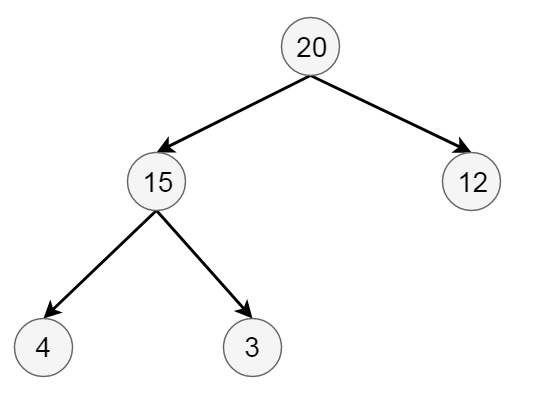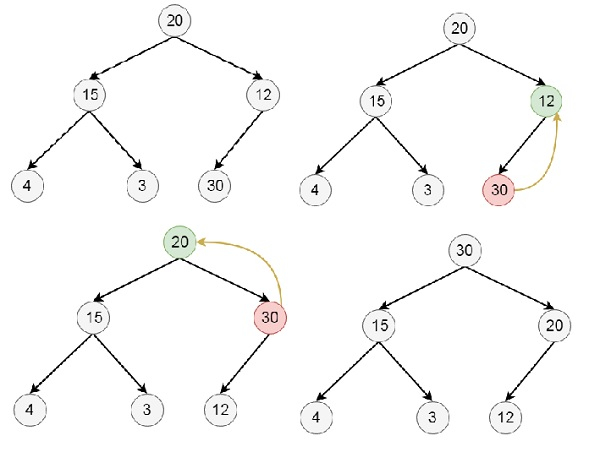# Insertion into a Max Heap in Data Structure

Here we will see how to insert and elements from binary max heap data structures. Suppose the initial tree is like below −## Insertion Algorithm

insert(heap, n, item) −
Begin
if heap is full, then exit
else
n := n + 1
for i := n, i > 1, set i := i / 2 in each iteration, do
if item <= heap[i/2], then break
heap[i] = heap[i/2]
done
end if
heap[i] := item
End

## Example

Suppose we want to insert 30 into the heap −